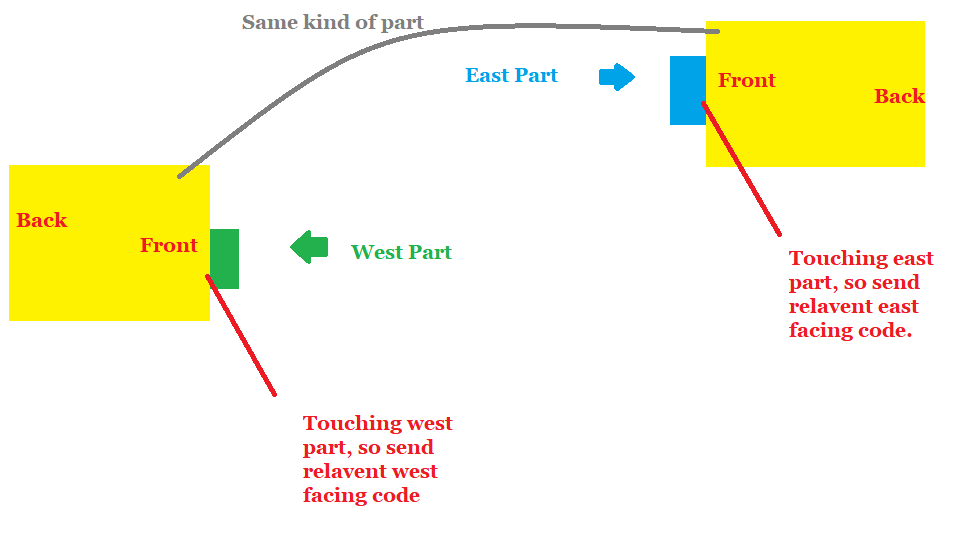# How to check if a part is facing a direction

Hello all,

I’m trying to make an if statement that checks if a part is facing a certain direction. How would I do this?

Is there a property that I can check?

``````-- example
if part.WhatWouldGoHere = Value then
game.Workspace.Part.Color = 'Red'
end
``````
4 Likes
``````local lookVector = part.CFrame.LookVector

local up = Vector3.new(0, 1, 0);
local down = Vector3.new(0, -1, 0);
local left = Vector3.new(-1, 0, 0);
local right = Vector3.new(1, 0, 0);
local forward = Vector3.new(0, 0, -1);
local back = Vector3.new(0, 0, 1);

local upDot = lookVector:Dot(up)
local downDot = lookVector:Dot(down)
local leftDot = lookVector:Dot(left)
local rightDot = lookVector:Dot(right)
local forwardDot = lookVector:Dot(forward)
local backDot = lookVector:Dot(back)

local facingDot = math.max(upDot, downDot, leftDot, rightDot, forwardDot, backDot)

if facingDot == upDot then
print("Facing up")
elseif facingDot == downDot then
print("Facing down")
-- elseif facingDot == rightDot then -- etc.
end
``````
1 Like

Or just do `if direction:Dot(part.CFrame.LookVector) > 0 then`

1 Like

This is a lot of unnecessary code that does not suite this use case.

2 Likes

It is perfectly necessary; it checks every direction and compares that Dot product to the look vector of the given part’s CFrame to see which direction in world space that it is closest.

Which can be done in one line of code if hes only looking for comparing to a single direction
Which luckily he is

1 Like

Yes, I gave him an entire general purpose function that accomplishes this task as well.

Simplicity is beautiful and less hard allowing shorter and easier to understand scripts

2 Likes

And if you want to check if the part is facing in a certain direction by how many degrees, use this:

``````local desiredDirection = Vector3.new(0, 1, 0)
local currentPartDirection = part.CFrame.LookVector

local angleInDegrees = math.deg(math.acos(math.clamp(desiredDirection:Dot(currentPartDirection), -1, 1)))

if angleInDegrees < 30 then
print("Part is facing towards target within a 30 degree radius!")
end``````
1 Like
``````
local part = script.Parent

part.Velocity = part.CFrame.LookVector * 30
``````

look vector is a value of CFrame. It checks where the part’s front surface is looking. if that’s what your looking for, here’s your answer.

This is a more unorthodox way of doing this, but you could try it like this, you could set parts that are set as west east etc. And you could send relevant code when it senses which part it is. The code up above is a much better way to do it, but this is possibly an easier route.I accidentally spelled relevant wrong1 Like

Good idea, but what about parts that are facing across from each other?

1 Like

I am pretty sure it should still work. I might be wrong though.

Thanks for the reply. I have a slightly simpler way of using a different method to solve the problem, but thank you for helping!

1 Like

Ok nice. It if is no problem, how did you do it?